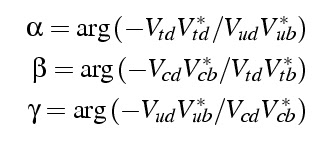## Monday, December 24, 2007

### The Unitary Triangle[The Unitary Triangle, by the CKMfitter Group]

My husband and I, we both agreed the Unitary Triangle above is the prettiest plot of contemporary physics. Unfortunately, it turns out neither of us knows very much about the actual experiments that constrain the parameter space, so we'll have to be a bit brief in this regard and just refer you elsewhere for the details.

The quantities constrained in this plot are parameters of the Cabibbo-Kobayashi-Maskawa matrix, VCKM, which maps the weak eigenstates of the quarks to the mass eigenstates. This matrix it not diagonal, and mixes quark flavors in a similar way how the neutrino mixing works.Here, the primes indicate the weak eigenstates. For example, it is the d' quark that transforms into an up quark in the weak decay mediated by the charged W boson - this is, on the quark level, the process which is going on in the radioactive decay of the neutron into a proton, an electron, and an antineutrino. The CKM matrix is unitary, which means the elements have to fulfil the relationand other similar relations, but this is the one frequently used. This is a sum of three complex numbers that add up to zero. One can draw these numbers as vectors in the complex plane, and since they sum up to zero they will have to form a closed triangle. The relative angles between two sides are the arguments of the ratios of these complex numbersSince it's a triangle one has α+β+γ = π. In addition, one can normalize one of the sides to length one and turn it such that it lies on the real axis. The resulting triangle is what you see in the plot above, the task is now to constrain it's shape, i.e. the angles or side lengths respectively. The entries of the CKM matrix affect a lot of particle physics processes, so one has to figure out smart ways how to extract them from the data. Most experiments look in detail at the weak decay of hadrons such as Kaons and B mesons. In these decays, strange and bottom quarks decay into up and charm quarks, respectively. Moreover, some of the experiments involve penguins.

Some experiments constrain an angle with a certain precision - this you see in the plot as the light blue shaded outgoing beams. Different shades indicate confidence levels. The angle β is studied through the interference between decays of neutral B0 mesons; γ is more challenging to measure and requires studies of rare decay processes; and also α is difficult to measure because quantum effects interfere with direct measurements. Other experiments constrain the length of a side which results in the green and orange circles. The triangle’s left side is measured through the decay rate of bottom quarks into up and charm quark; the triangle’s right side is constrained by the rate the B0 meson spontaneously turns into its antiparticle. And then there is the boomerang shaped green region which results from measurements of CP violation in Kaon decays.

Taken together this data constrains the top of the diagram to lie in the region marked with the red boundary.

The CKM matrix is one of the less beautiful parts of the standard model of particle physics, despite the aesthetic appeal of the unitary triangle. There are no explanations for the entries of the matrix, which just have to be taken as some parameters of the standard model. Nevertheless, the CKM matrix is relevant to quantify CP violation, which helps to understand the predominance of matter over antimatter in the universe.

This post is part of our 2007 advent calendar A Plottl A Day.

1.Bee,

I thought at first that this was one of your paintings!!

2.Perhaps the most interesting aspect of the CKM matrix in the standard model is that it **cannot** explain the matter/anti-matter asymmetry of the universe. The level of CP violation indicated by the measurements depicted in this very pretty plot is simply too small...

PS. The "plot a day" was a wonderful idea!

COMMENTS ON THIS BLOG ARE PERMANENTLY CLOSED. You can join the discussion on Patreon.